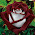## Friday, February 27, 2015

### 42 | Aldridge Murder Spree in Missouri

This story has much to do with '42'.
• February = 6+5+2+9+3+1+9+7 = 42
• Missouri = 4+9+1+1+6+3+9+9 = 42
• Aldridge = 1+3+4+9+9+4+7+5 = 42
This story is full of numerology, including the 74-year old mother.
• Joseph = 1+6+1+5+7+8 = 28/37
• Jesse = 1+5+1+1+5 = 13/31
• Aldridge = 1+3+4+9+9+4+7+5 = 42
• Joseph Jesse Aldridge = 83/110

#### 1 comment:

1.If you are calculating the numbers, especially to see if it is a possible psyop, you should use the system the ones in the shadows use: the Chaldean-Hebrew Kabbalah method.

Joesph = 29/11/2
* His first name = Grace Under Pressure
Joe = 13/4
* Nicknamed = Death/Change/Regeneration
Jesse = 17/8
Aldridge = 23/5
Joseph Jesse Aldridge = 15/6
* His full name = The Magician
Joe Aldridge = 9 (27)
* The name he went by = Mars (It does not add up to a compound number in itself.)

26 = Partnership

02.26.2015 = 18/9 = Spiritual/Material Conflict

02.26.15 = 16/7 = Shattered Citadel